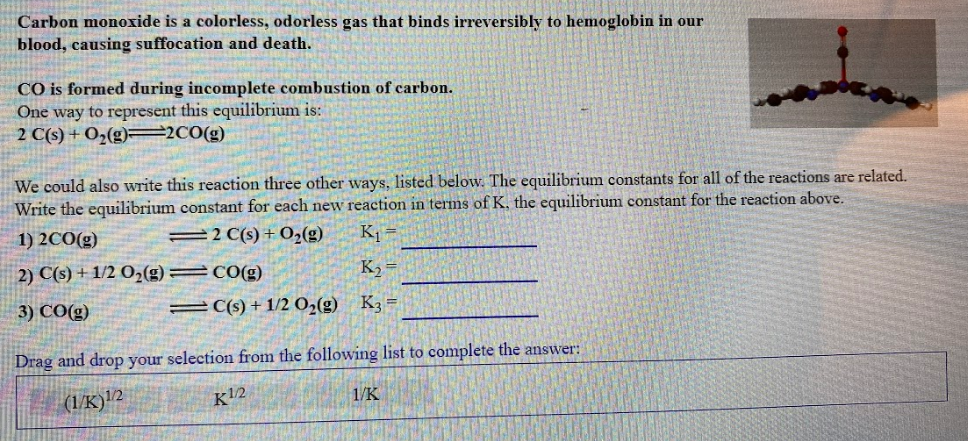# Carbon monoxide is a colorless, odorless gas that binds irreversibly to hemoglobin in our blood, causing suffocation and death. CO is formed during incomplete combustion of carbon. One way to represent this equilibrium is: 2 C(s) + O2(g) ⇌ 2CO(g) We could also write this reaction three other ways, listed below. The equilibrium constants for all of the reactions are related. Write the equilibrium constant for each new reaction in terms of K, the equilibrium constant for the reaction above. 1) 2CO(g) ⇌ 2C(s) + O2(g) K1 = _____ 2) C(s) + 1/2 O2(g) ⇌ CO(g) K2 = _____ 3) CO(g) ⇌ C(s) + 1/2 O2(g) K3 = _____